Documentation

wthrmngr

Threshold settings manager

Description

wthrmngr returns a global threshold or level-dependent thresholds for wavelet-based denoising and compression. The function derives thresholds from the wavelet coefficients in a wavelet decomposition.

The thresholds are used by Wavelet Toolbox™ denoising and compression tools, such as command-line functions and the Wavelet Analyzer app.

example

thr = wthrmngr(opt,method,C,L) returns the threshold for the [C,L] wavelet decomposition of the signal or image to compress or denoise. For signals, [C,L] is the output of wavedec. For images, [C,L] is the output of wavedec2.

example

thr = wthrmngr(opt,method,C,L,alpha) returns the [C,L] wavelet decomposition threshold using the sparsity parameter alpha. For signals, [C,L] is the output of wavedec. For images, [C,L] is the output of wavedec2.

To learn more about alpha, see wdcbm or wdcbm2 for compression, and wbmpen for denoising.

thr = wthrmngr(opt,method,C,L,scale) returns the [C,L] wavelet decomposition threshold using the type of multiplicative threshold rescaling specified in scale. For signals, [C,L] is the output of wavedec. For images, [C,L] is the output of wavedec2.

The 'rigrsure', 'heursure', and 'minimaxi' denoising methods are only applicable to signals.

thr = wthrmngr(opt,method,swtdec,alpha) returns the level-dependent threshold for the stationary wavelet decomposition, swtdec, of the signal or image to denoise. alpha specifies the sparsity parameter (see wbmpen). For signals, swtdec is the output of swt. For images, swtdec is the output of swt2.

Thresholds are derived from a subset of the coefficients in the stationary wavelet decomposition. For more information, see Coefficient Selection.

example

thr = wthrmngr(opt,method,swtdec,scale) returns the level-dependent threshold for the stationary wavelet decomposition using the type of multiplicative threshold rescaling specified in scale. For signals, swtdec is the output of swt. For images, swtdec is the output of swt2.

Thresholds are derived from a subset of the coefficients in the stationary wavelet decomposition. For more information, see Coefficient Selection.

The 'rigrsure', 'heursure', and 'minimaxi' denoising methods apply only to signals.

example

thr = wthrmngr(opt,method,wpt) returns the global threshold for the wavelet packet decomposition, wpt, of the signal or image to compress or denoise.

thr = wthrmngr(opt,'rem_n0',X) returns the global threshold to compress the signal or image, X, using the specified wavelet option and method 'rem_n0'.

If opt is 'dw1dcompGBL' or 'dw2dcompGBL', thresholds are based on the finest-scale wavelet coefficients obtained using the Haar wavelet. If opt is 'wp1dcompGBL' or 'wp2dcompGBL', thresholds are based on the finest-scale wavelet packet coefficients obtained using the Haar wavelet.

Examples

collapse all

Load and plot a noisy signal.

plot(noisdopp)
grid on
title('Noisy Signal')Generate a level 5 wavelet decomposition of the noisy signal using the order 4 Daubechies wavelet. Plot the coefficients.

[c,l] = wavedec(noisdopp,5,'db4');
plot(c)
grid on
title('Wavelet Coefficients')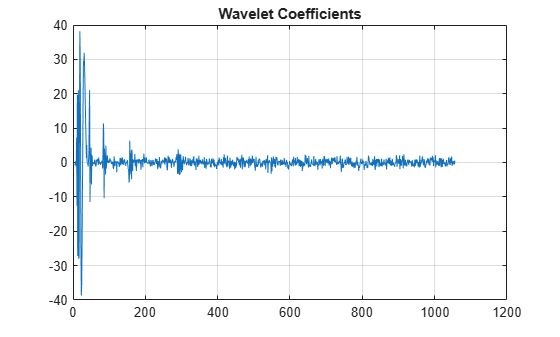Determine a global threshold for compressing the signal.

thr = wthrmngr('dw1dcompGBL','bal_sn',c,l);

The index of the first wavelet detail coefficient in c is l(1)+1. Apply the threshold to all the detail coefficients. Plot the thresholded coefficients. Observe that most of the coefficients have been set to 0.

c(l(1)+1:end) = c(l(1)+1:end).*(c(l(1)+1:end)>thr);
plot(c)
grid on
title('Thresholded Coefficients')Reconstruct the signal from the thresholded coefficients. Plot the reconstruction.

xrec = waverec(c,l,'db4');
plot(xrec)
grid on
title('Compressed Signal')Compress an image using the Birgé-Massart strategy.

Load an image and add white Gaussian noise. For purposes of reproducibility, set the random seed to the default value.

rng default
x = X+18*randn(size(X));

Obtain the 2-D discrete wavelet transform down to level 3 using the Daubechies least-asymmetric wavelet with 4 vanishing moments. Obtain the compression thresholds using the Birgé-Massart strategy with sparsity parameter, alpha, equal to 2.

[C,L] = wavedec2(x,3,'sym4');
alpha = 2;
THR = wthrmngr('dw2dcompLVL','scarcehi',C,L,alpha);

Compress the image and display the result.

xd = wdencmp('lvd',x,'sym4',3,THR,'s');
image(X)
title('Original Image')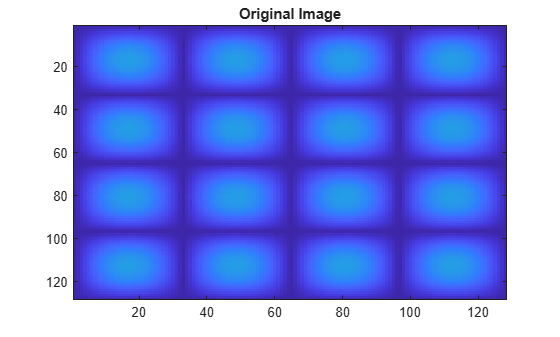figure
image(x)
title('Noisy Image')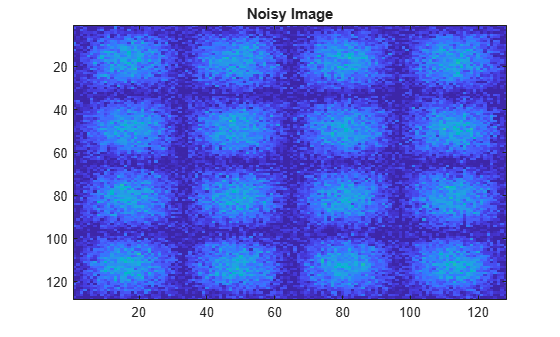figure
image(xd)
title('Compressed Image')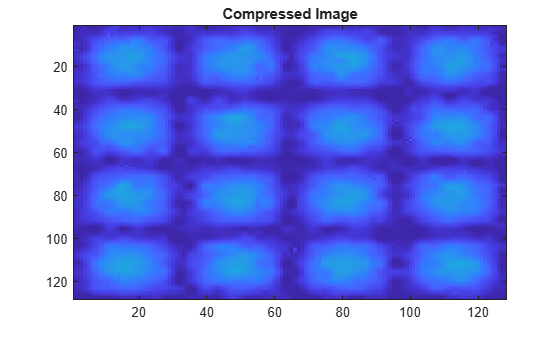This example uses a level-dependent threshold derived from the wavelet coefficients at each scale to implement hard thresholding with the stationary wavelet transform.

Load the noisy blocks signal. Obtain the stationary wavelet transform down to level 5 by using the Haar wavelet.

L = 5;
swc = swt(noisbloc,L,'haar');

Make a copy of the wavelet transform coefficients. Determine the Donoho-Johnstone universal threshold based on the detail coefficients for each scale. Using the 'mln' option, wthrmngr returns a 1-by-L vector, with every element equal to the universal threshold for the corresponding scale.

swcnew = swc;
ThreshML = wthrmngr('sw1ddenoLVL','sqtwolog',swc,'mln');

Use the universal thresholds to implement hard thresholding. The thresholds are applied in a scale-dependent manner.

for jj = 1:L
swcnew(jj,:) = wthresh(swc(jj,:),'h',ThreshML(jj));
end

Invert the stationary wavelet transform on the thresholded coefficients, swcnew. Plot the original signal and the denoised signal for comparison.

noisbloc_denoised = iswt(swcnew,'haar');
plot(noisbloc)
hold on
plot(noisbloc_denoised,'r','linewidth',2)
legend('Original','Denoised')Denoise a noisy signal by applying a global threshold to a wavelet packet decomposition structure.

Load and plot a noisy signal.

plot(noisdopp)
grid on
title('Noisy Signal')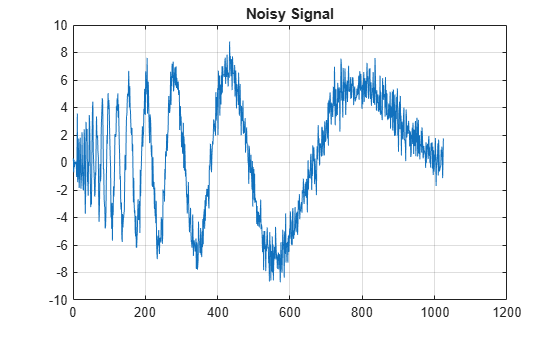Generate a level 3 wavelet packet decomposition of the noisy signal using the order 4 Daubechies wavelet.

T = wpdec(noisdopp,3,'db4');

Determine a global threshold for denoising the signal.

thr = wthrmngr('wp1ddenoGBL','sqtwologuwn',T);

Obtain the leaves from the wavelet packet decomposition tree T and apply the threshold to the leaves. Use hard thresholding.

T1 = T;
sorh = 'h';
cfs = wthresh(cfs,sorh,thr);
T1 = write(T1,'data',cfs);

Reconstruct the denoised signal from the thresholded coefficients. Plot the reconstruction.

xrec = wprec(T1);
plot(xrec)
grid on
title('Denoised Signal')This example uses a level-independent threshold based on the finest-scale wavelet coefficients to implement hard thresholding with the stationary wavelet transform.

Load the noisy blocks signal. Obtain the stationary wavelet transform down to level 5 by using the Haar wavelet.

L = 5;
swc = swt(noisbloc,L,'haar');

Make a copy of the wavelet transform coefficients. Determine the Donoho-Johnstone universal threshold based on the first-level detail coefficients. Using the 'sln' option, wthrmngr returns a 1-by-L vector, with every element equal to the same value. Take the mean of the vector to obtain a scalar threshold.

swcnew = swc;
ThreshSL = mean(wthrmngr('sw1ddenoLVL','sqtwolog',swc,'sln'));

Use the universal threshold to implement hard thresholding. The same threshold is applied to the wavelet coefficients at every level.

for jj = 1:L
swcnew(jj,:) = wthresh(swc(jj,:),'h',ThreshSL);
end

Invert the stationary wavelet transform on the thresholded coefficients, swcnew. Plot the original signal and the denoised signal for comparison.

noisbloc_denoised = iswt(swcnew,'haar');
plot(noisbloc)
hold on
plot(noisbloc_denoised,'r','linewidth',2)
legend('Original','Denoised')Input Arguments

collapse all

Type and dimension of compression or denoising, specified as one of the values listed in the tables that follow. wthrmngr returns thresholds appropriate for the option you specify.

With a discrete wavelet or wavelet packet decomposition of the data, you can compress or denoise that data. With a stationary wavelet decomposition of the data, you can only denoise the data.

For an explanation of which coefficients are used to determine the thresholds, see Coefficient Selection.

1-D Discrete Wavelet Decomposition Options

In these options, X is the signal, the wavelet coefficients are in the vector C, and the lengths of the coefficient vectors are in L. The argument alpha is the sparsity parameter, and scale defines the multiplicative threshold rescaling.

For additional information regarding the wavelet decomposition, see wavedec. To learn more about alpha and scale, see wdcbm and wden respectively.

opt

Description

Valid Syntaxes
'dw1dcompGBL'

1-D compression using a global threshold

• thr = wthrmngr('dw1dcompGBL','rem_n0',X)

• thr = wthrmngr('dw1dcompGBL','bal_sn',C,L)

'dw1dcompLVL'

1-D compression using level-dependent thresholds

• thr = wthrmngr('dw1dcompLVL','scarcehi',C,L,alpha), where 2.5 < alpha < 10

• thr = wthrmngr('dw1dcompLVL','scarceme',C,L,alpha), where 1.5 < alpha < 2.5

• thr = wthrmngr('dw1dcompLVL','scarcelo',C,L,alpha), where 1 < alpha < 2

'dw1ddenoLVL'

1-D denoising using level-dependent thresholds

• thr = wthrmngr('dw1ddenoLVL','sqtwolog',C,L,scale)

• thr = wthrmngr('dw1ddenoLVL','rigrsure',C,L,scale)

• thr = wthrmngr('dw1ddenoLVL','heursure',C,L,scale)

• thr = wthrmngr('dw1ddenoLVL','minimaxi',C,L,scale)

• thr = wthrmngr('dw1ddenoLVL','penalhi',C,L,alpha), where 2.5 < alpha < 10

• thr = wthrmngr('dw1ddenoLVL','penalme',C,L,alpha), where 1.5 < alpha < 2.5

• thr = wthrmngr('dw1ddenoLVL','penallo',C,L,alpha), where 1 < alpha < 2

2-D Discrete Wavelet Decomposition Options

In these options, X is the data, the wavelet coefficients are in the vector C, and the size of the coefficient matrices are in L. The argument alpha is the sparsity parameter, and scale defines the multiplicative threshold rescaling.

For additional information regarding the wavelet decomposition, see wavedec2. To learn more about alpha and scale, see wdcbm2 and wden respectively.

opt

Description

Valid Syntaxes
'dw2dcompGBL'

2-D compression using a global threshold

• thr = wthrmngr('dw2dcompGBL','rem_n0',X)

• thr = wthrmngr('dw2dcompGBL','bal_sn',C,L)

• thr = wthrmngr('dw2dcompGBL','sqrtbal_sn',C,L)

'dw2dcompLVL'

2-D compression using level-dependent thresholds

• thr = wthrmngr('dw2dcompLVL','scarcehi',C,L,alpha), where 2.5 < alpha < 10

• thr = wthrmngr('dw2dcompLVL','scarceme',C,L,alpha), where 1.5 < alpha < 2.5

• thr = wthrmngr('dw2dcompLVL','scarcelo',C,L,alpha), where 1 < alpha < 2

'dw2ddenoLVL'

2-D denoising using level-dependent thresholds

• thr = wthrmngr('dw2ddenoLVL','sqrtbal_sn',C,L)

• thr = wthrmngr('dw2ddenoLVL','penalhi',C,L,alpha), where 2.5 < alpha < 10

• thr = wthrmngr('dw2ddenoLVL','penalme,C,L,alpha), where 1.5 < alpha < 2.5

• thr = wthrmngr('dw2ddenoLVL','penallo,C,L,alpha), where 1 < alpha < 2

• thr = wthrmngr('dw2ddenoLVL','sqtwolog',C,L,scale)

1-D Wavelet Packet Decomposition Options

In these options, X is the signal and wpt is the wavelet packet decomposition structure of the signal.

For additional information regarding the wavelet packet decomposition, see wpdec.

opt

Description

Valid Syntaxes
'wp1dcompGBL'

1-D compression using a global threshold

• thr = wthrmngr('wp1dcompGBL','rem_n0',X)

• thr = wthrmngr('wp1dcompGBL','bal_sn',wpt)

'wp1ddenoGBL'

1-D denoising using a global threshold

• thr = wthrmngr('wp1ddenoGBL','sqtwologuwn',wpt)

• thr = wthrmngr('wp1ddenoGBL','sqtwologswn',wpt)

• thr = wthrmngr('wp1ddenoGBL','bal_sn',wpt)

• thr = wthrmngr('wp1ddenoGBL','penalhi',wpt)

The wpbmpen function is used with the tuning parameter ALPHA = 6.25.

• thr = wthrmngr('wp1ddenoGBL','penalme',wpt)

The wpbmpen function is used with the tuning parameter ALPHA = 2.

• thr = wthrmngr('wp1ddenoGBL','penallo',wpt)

The wpbmpen function is used with the tuning parameter ALPHA = 1.5.

2-D Wavelet Packet Decomposition Options

In these options, X is the data and wpt is the wavelet packet decomposition structure of the data.

For additional information regarding the wavelet packet decomposition, see wpdec2.

opt

Description

Valid Syntaxes
'wp2dcompGBL'

2-D compression using a global threshold

• thr = wthrmngr('wp2dcompGBL','rem_n0',X)

• thr = wthrmngr('wp2dcompGBL','bal_sn',wpt)

• thr = wthrmngr('wp2dcompGBL','sqrtbal_sn',wpt)

'wp2ddenoGBL'

2-D denoising using a global threshold

• thr = wthrmngr('wp2ddenoGBL','sqtwologuwn',wpt)

• thr = wthrmngr('wp2ddenoGBL','sqtwologswn',wpt)

• thr = wthrmngr('wp2ddenoGBL','sqrtbal_sn',wpt)

• thr = wthrmngr('wp2ddenoGBL','penalhi',wpt)

The wpbmpen function is used with the tuning parameter ALPHA = 6.25.

• thr = wthrmngr('wp2ddenoGBL','penalme',wpt)

The wpbmpen function is used with the tuning parameter ALPHA = 2.

• thr = wthrmngr('wp2ddenoGBL','penallo',wpt)

The wpbmpen function is used with the tuning parameter ALPHA = 1.5.

1-D Stationary Wavelet Decomposition Options

Denoising using level-dependent thresholds is the only option available for a 1-D stationary wavelet decomposition, swtdec. In this option, alpha is a sparsity parameter and scale defines the multiplicative threshold rescaling.

For more information regarding the stationary wavelet decomposition, see swt. To learn more about alpha and scale, see wbmpen and wden respectively.

optValid Syntaxes
'sw1ddenoLVL'
• thr = wthrmngr('sw1ddenoLVL','sqtwolog',swtdec,scale)

• thr = wthrmngr('sw1ddenoLVL','rigrsure',swtdec,scale)

• thr = wthrmngr('sw1ddenoLVL','heursure',swtdec,scale)

• thr = wthrmngr('sw1ddenoLVL','minimaxi',swtdec,scale)

• thr = wthrmngr('sw1ddenoLVL','penalhi',swtdec,alpha), where 2.5 < alpha < 10

• thr = wthrmngr('sw1ddenoLVL','penalme',swtdec,alpha), where 1.5 < alpha < 2.6

• thr = wthrmngr('sw1ddenoLVL','penallo',swtdec,alpha), where 1 < alpha < 2

Thresholds are based on a subset of the coefficients in the stationary wavelet decomposition. See Coefficient Selection for additional information.

2-D Stationary Wavelet Decomposition Options

Denoising using level-dependent thresholds is the only option available for a 2-D stationary wavelet decomposition, swtdec. In this option, alpha is a sparsity parameter and scale defines the multiplicative threshold rescaling.

For more information regarding the stationary wavelet decomposition, see swt2. To learn more about alpha and scale, see wbmpen and wden respectively.

optValid Syntaxes
'sw2ddenoLVL'
• thr = wthrmngr('sw2ddenoLVL','sqrtbal_sn',swtdec)

• thr = wthrmngr('sw2ddenoLVL','penalhi',swtdec,alpha) where 2.5 < alpha < 10

• thr = wthrmngr('sw2ddenoLVL','penalme',swtdec,alpha) where 1.5 < alpha < 2.5

• thr = wthrmngr('sw2ddenoLVL','penallo',swtdec,alpha) where 1 < alpha < 2

• thr = wthrmngr('sw2ddenoLVL','sqtwolog',swtdec,scale)

Thresholds are based on a subset of the coefficients in the stationary wavelet decomposition. See Coefficient Selection for additional information.

Thresholding method, specified as one of the values listed here.

methodDescription
'scarcehi'Uses Birgé-Massart strategy for determining thresholds.
'scarceme'Uses Birgé-Massart strategy for determining thresholds.
'scarcelo'Uses Birgé-Massart strategy for determining thresholds.
'sqtwolog'Uses fixed-form universal threshold. See 'sqtwolog' option in wden.
'sqtwologuwn'Uses fixed-form universal threshold. See 'sqtwolog' option in wden when used with 'sln' option.
'sqtwologswn'Uses fixed-form universal threshold. See 'sqtwolog' option in wden when used with 'mln' option.
'rigsure'Uses soft threshold estimator rule based on Stein's Unbiased Estimate of Risk. See 'SURE' option in wdenoise.
'heursure'Uses mixture of 'rigsure' and 'sqtwolog'. See 'heursure' option in wden.
'minimaxi' Uses a fixed threshold chosen which yields minimax performance. See 'Minimax' option in wdenoise.
'penalhi'Used to define Birgé-Massart strategy for determining thresholds.
'penalme'Used to define Birgé-Massart strategy for determining thresholds.
'penallo'Used to define Birgé-Massart strategy for determining thresholds.
'rem_n0'Returns a threshold close to 0. A typical THR value is median(abs(coefficients)).
'bal_sn'Returns a threshold such that the percentages of retained energy and number of zeros are the same.
'sqrtbal_sn'Returns a threshold equal to the square root of the value such that the percentages of retained energy and number of zeros are the same.

Data Types: char

Input data, specified as a real-valued vector or real-valued matrix.

Data Types: double

Wavelet expansion coefficients of the data to be compressed or denoised, specified as a real-valued vector. If the data is one-dimensional, C is the output of wavedec. If the data is two-dimensional, C is the output of wavedec2.

Example: [C,L] = wavedec(randn(1,1024),3,'db4')

Data Types: double

Size of wavelet expansion coefficients of the signal or image to be compressed or denoised, specified as a vector or matrix of positive integers.

For signals, L is the output of wavedec. For images, L is the output of wavedec2.

Example: [C,L] = wavedec(randn(1,1024),3,'db4')

Data Types: double

Sparsity parameter used for compressing or denoising data, specified as a positive scalar greater than 1 and less than 10. See wdcbm, wdcbm2, and wbmpen for additional information.

Data Types: double

Multiplicative threshold rescaling, specified as one of the following:

• 'one' — No rescaling

• 'sln' — Rescaling using a single estimation of level noise based on first-level coefficients

• 'mln' — Rescaling using a level-dependent estimation of level noise

Stationary wavelet decomposition structure of data to be compressed or denoised, specified as a real-valued matrix. If the data is one-dimensional, swtdec is the output of swt. If the data is two-dimensional, swtdec is the output of swt2.

Example: swtdec = swt2(randn(256),3,'db1')

Data Types: double

Wavelet packet decomposition structure of the data to be compressed or denoised. If the data is one-dimensional, wpt is the output of wpdec. If the data is two-dimensional, wpt is the output of wpdec2.

Example: wpt = wpdec(randn(1,1024),5,'db1')

Output Arguments

collapse all

Threshold, returned as a real-valued scalar for global thresholds, or a real-valued vector or matrix for level-dependent thresholds.

Data Types: double

Tips

• To denoise 1-D signals, consider using the Wavelet Signal Denoiser. The app visualizes and denoises real-valued 1-D signals using default parameters. You can also compare results. In addition, you can also recreate the denoised signal in your workspace by generating a MATLAB® script, which uses the wdenoise function.

Algorithms

collapse all

Coefficient Selection

A critically sampled wavelet or wavelet packet decomposition involves decimating coefficients by a factor of 2 at each stage of the decomposition. Decimation does not occur in the nondecimated stationary wavelet decomposition.

wthrmngr derives denoising and compression thresholds from the wavelet coefficients. For a critically sampled wavelet or wavelet packet decomposition, the option and method determine whether all wavelet coefficients or only the finest scale coefficients are used.

For the stationary wavelet decomposition, wthrmngr always uses a subset of the wavelet coefficients. When computing the denoising thresholds of an N-level stationary wavelet decomposition, the algorithm first subsamples the wavelet coefficients at level k by a factor of 2k, for k = 1,…,N. The algorithm uses this subset of coefficients to determine the thresholds. Most of the coefficients in the stationary wavelet decomposition are not considered.

Birgé-Massart Strategy

The Birgé-Massart strategy for determining thresholds depends on several different parameters. You specify the wavelet decomposition and a thresholding method. You can also specify a sparsity parameter, alpha, or a specific multiplicative threshold rescaling, scale. Based on your inputs, wthrmngr derives the necessary Birgé-Massart parameters. The parameters depend on the dimension of the signal, and the total number, N, of coefficients at the coarsest scale of wavelet decomposition.

If the thresholding method is 'scarcehi', 'scarceme', or 'scarcelo', the wthrmngr executes either wdcbm or wdcbm2. If the thresholding method is 'penalhi', 'penalme', or 'penallo', then wthrmngr executes either wbmpen or wpbmpen.

Thresholding Method

Description

'scarcehi'
• If the signal is 1-D, then wdcbm is used with input argument M = N.

• If the signal is 2-D, then wdcbm2 is used with M = 4*N.

'scarceme'
• If the signal is 1-D, then wdcbm is used with input argument M = 3*N/2.

• If the signal is 2-D, then wdcbm2 is used with input argument with M = 16*N/3.

'scarcelo'
• If the signal is 1-D, then wdcbm is used with input argument M = 2 N.

• If the signal is 2-D, then wdcbm2 is used with input argument M = 32*N/3.

'penalhi'
• If the input is a wavelet decomposition, then wbmpen is used with ALPHA = 5*(3*alpha+1)/8.

• If the input is a wavelet packet decomposition, then wpbmpen is used ALPHA = 6.25.

'penalme'
• If the input is a wavelet decomposition, then wbmpen is used with ALPHA = (alpha+5)/8.

• If the input is a wavelet packet decomposition, then wpbmpen is used ALPHA = 2.

'penallo'
• If the input is a wavelet decomposition, then wbmpen is used with ALPHA = (alpha+3)/4.

• If the input is a wavelet packet decomposition, then wpbmpen is used ALPHA = 1.5.

 Birgé, L., and P. Massart. “From Model Selection to Adaptive Estimation.” Festschrift for Lucien Le Cam: Research Papers in Probability and Statistics (E. Torgersen, D. Pollard, and G. Yang, eds.). New York: Springer-Verlag, 1997, pp. 55–88.

Wavelet Toolbox DocumentationGet trial now